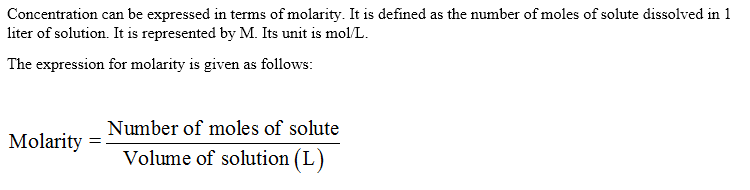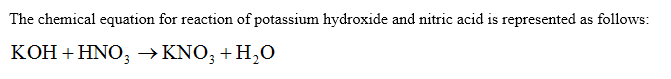# 25.13 mL of 0.250 M KOH is required to neutralize 10.00 mL of nitric acid. What is the concentration of nitric acid?

Question
66 views

25.13 mL of 0.250 M KOH is required to neutralize 10.00 mL of nitric acid. What is the concentration of nitric acid?

check_circle

Step 1

Volume of KOH (V1) = 25.13 mL

Molarity of KOH solution (M1) = 0.250 M

Volume of HNO3 solution (V2) = 10.00 mL

Step 2Step 3...

### Want to see the full answer?

See Solution

#### Want to see this answer and more?

Solutions are written by subject experts who are available 24/7. Questions are typically answered within 1 hour.*

See Solution
*Response times may vary by subject and question.
Tagged in